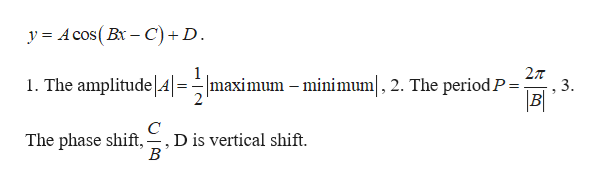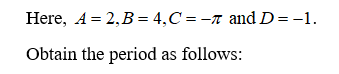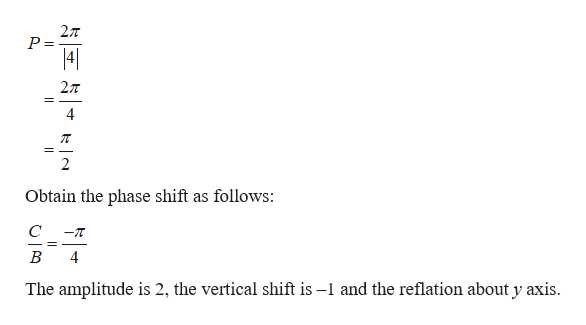# State amplitude,reflection,period,vertical translation and phase shift for each function and graph.y=-1+2cos(4x+pi)

Question
94 views

State amplitude,reflection,period,vertical translation and phase shift for each function and graph.

y=-1+2cos(4x+pi)

check_circle

Step 1

Note that, the general form ishelp_outlineImage Transcriptioncloseу%3D Аcos( Bx —С) + D. 2л 1. The amplitude A 2 maximum minimum|, 2. The period.P= 3 B The phase shift, D is vertical shift. В fullscreen
Step 2

The given function is

Step 3...help_outlineImage Transcriptionclose2л P = 41 = 4 Obtain the phase shift as follows: C -T В 4 The amplitude is 2, the vertical shift is -1 and the reflation about y axis fullscreen

### Want to see the full answer?

See Solution

#### Want to see this answer and more?

Solutions are written by subject experts who are available 24/7. Questions are typically answered within 1 hour.*

See Solution
*Response times may vary by subject and question.
Tagged in

### Other